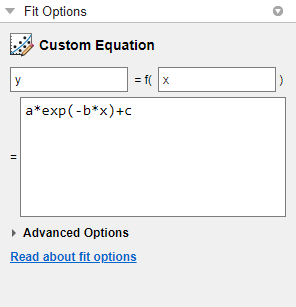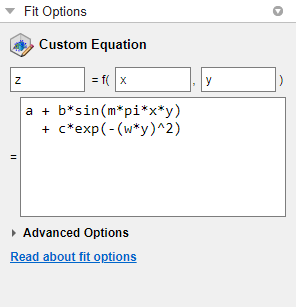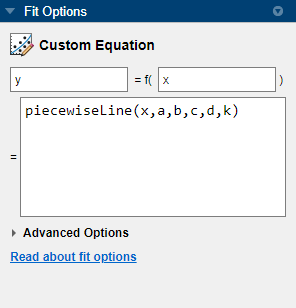## Custom Models

### Custom Models vs. Library Models

If the toolbox library does not contain a desired parametric equation, you can create your own custom equation. Library models, however, offer the best chance for rapid convergence. This is because:

• For most library models, the toolbox calculates optimal default coefficient starting points. For custom models, the toolbox chooses random default starting points on the interval [0,1]. You need to find suitable start points for custom models.

• Library models use an analytic Jacobian. Custom models use finite differencing.

#### Linear and Nonlinear Fitting

You can create custom general equations with the Custom Equation fit type. General models are nonlinear combinations of (perhaps nonlinear) terms. They are defined by equations that might be nonlinear in the parameters. The custom equation fit uses the nonlinear least-squares fitting procedure.

You can define a custom linear equation using the Custom Equation fit type, though the nonlinear fitting is less efficient and usually slower than linear least-squares fitting.

• If you don’t know if your equation can be expressed as a set of linear functions, then select `Custom Equation`. You might need to search for suitable start points.

• If you need linear least-squares fitting for custom equations, select the `Linear Fitting` model type instead. See Custom Linear Fitting.

### Selecting a Custom Equation Fit Interactively

In the Curve Fitting app, select `Custom Equation` from the model type list.

Use the custom equation fit to define your own equations. An example custom equation appears when you select Custom Equation from the list, as shown here for curve data.If you have surface data, the example custom equation uses both x and y.1. You can edit `x`, `y`, and `z` to any valid variable names.

2. In the lower box, edit the example to define your own custom equation. You can enter any valid MATLAB® expression in terms of your variable names. You can specify a function or script name (see Fitting a Curve Defined by a File in the Curve Fitting App).

3. Click if you want to specify start points or bounds. By default, the starting values are randomly selected on the interval [0,1] and are unconstrained. You might need to search for suitable start points and bounds. For an example, see Custom Nonlinear ENSO Data Analysis.

If you set fit options and then alter other fit settings, the app remembers your choices for lower and upper bounds and start points, if possible. For custom equations Curve Fitting app always remembers user values, but for many library models if you change fit settings then the app automatically calculates new best values for start points or lower bounds.

You can save your custom equations as part of your saved Curve Fitting app sessions.

Your function can execute a number of times, both during fitting and during preprocessing before fitting. Be aware this may be time-consuming if you are using functions with side effects such as writing data to a file, or displaying diagnostic information to the Command Window.

For examples, see:

#### Fitting a Curve Defined by a File in the Curve Fitting App

This example shows how to provide a function or script name as the fitting model in the Curve Fitting app. Define a function in a file and use it to fit a curve.

1. Define a function in a MATLAB file.

```function y = piecewiseLine(x,a,b,c,d,k) % PIECEWISELINE A line made of two pieces % that is not continuous. y = zeros(size(x)); % This example includes a for-loop and if statement % purely for example purposes. for i = 1:length(x) if x(i) < k, y(i) = a + b.* x(i); else y(i) = c + d.* x(i); end end end```

Save the file on the MATLAB path.

2. Define some data and open the Curve Fitting app.

```x = [0.81;0.91;0.13;0.91;0.63;0.098;0.28;0.55;... 0.96;0.96;0.16;0.97;0.96]; y = [0.17;0.12;0.16;0.0035;0.37;0.082;0.34;0.56;... 0.15;-0.046;0.17;-0.091;-0.071]; cftool```
3. In the Curve Fitting app, select `x` and `y` in the X data and Y data lists.

4. Use your `piecewiseLine` function in the Curve Fitting app by selecting the Custom Equation fit type, and then entering your function expression in the custom equation text box. The function takes x data and some parameters for fitting.

`piecewiseLine( x, a, b, c, d, k )`

The Curve Fitting app creates a fit using your function.Tip

If you want to use the same function for fitting at the command line, use the same expression as an input to `fittype`, and then use the `fittype` as an input to `fit`:

```ft = fittype('piecewiseLine( x, a, b, c, d, k )'); f = fit( x, y, ft)```
For more examples, see the `fit` function.

### Selecting a Custom Equation Fit at the Command Line

To fit custom models, either:

• Supply a custom model to the `fit` function in the `fitType` input argument. You can use a MATLAB expression (including any `.m` file), a cell array of linear model terms, or an anonymous function.

• Create a `fittype` object with the `fittype` function to use as an input argument for the `fit` function.

This example loads some data and uses a custom equation defining a Weibull model as an input to the `fit` function:

```time = [ 0.1; 0.1; 0.3; 0.3; 1.3; 1.7; 2.1; 2.6; 3.9; 3.9; ... 5.1; 5.6; 6.2; 6.4; 7.7; 8.1; 8.2; 8.9; 9.0; 9.5; ... 9.6; 10.2; 10.3; 10.8; 11.2; 11.2; 11.2; 11.7; 12.1; 12.3; ... 12.3; 13.1; 13.2; 13.4; 13.7; 14.0; 14.3; 15.4; 16.1; 16.1; ... 16.4; 16.4; 16.7; 16.7; 17.5; 17.6; 18.1; 18.5; 19.3; 19.7;]; conc = [0.01; 0.08; 0.13; 0.16; 0.55; 0.90; 1.11; 1.62; 1.79; 1.59; ... 1.83; 1.68; 2.09; 2.17; 2.66; 2.08; 2.26; 1.65; 1.70; 2.39; ... 2.08; 2.02; 1.65; 1.96; 1.91; 1.30; 1.62; 1.57; 1.32; 1.56; ... 1.36; 1.05; 1.29; 1.32; 1.20; 1.10; 0.88; 0.63; 0.69; 0.69; ... 0.49; 0.53; 0.42; 0.48; 0.41; 0.27; 0.36; 0.33; 0.17; 0.20;]; f = fit( time, conc, 'c*a*b*x^(b-1)*exp(-a*x^b)', 'StartPoint', [0.01, 2, 5] ) plot( f, time, conc )```

To define a custom model using `fittype`, use the form:

`f = fittype(expr)`
which constructs a custom model `fittype` object for the MATLAB expression contained in the string, cell array, or anonymous function `expr`.

See the `fittype` reference page for details on:

• Specifying dependent and independent variables, problem parameters, and coefficients using `fittype`.

• Specifying a cell array of terms to use a linear fitting algorithm for your custom equation. If `expr` is a string or anonymous function, then the toolbox uses a nonlinear fitting algorithm.

For more details on linear fitting, see Selecting Linear Fitting at the Command Line.

• Examples of linear and nonlinear custom models.

For a step-by-step example, see Custom Nonlinear Census Fitting.